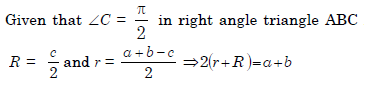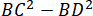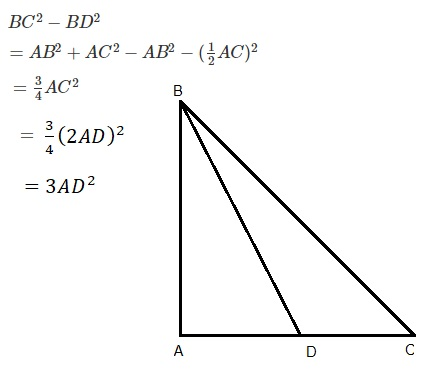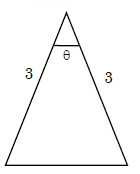## Aspire's Library

A Place for Latest Exam wise Questions, Videos, Previous Year Papers,
Study Stuff for MCA Examinations

## NIMCET Previous Year Questions (PYQs)

#### NIMCET Properties Of Triangle PYQ

NIMCET PYQ

If $\Delta=a^2-(b-c)^2$, where $\Delta$ is the are of the triangle ABC, then $tanA=$

NIMCET Previous Year PYQNIMCET NIMCET 2019 PYQ

#### Solution

NIMCET PYQ
In a triangle ABC, if the tangent of half the difference of two angles is equal to one third of the tangent of half the sum of the angles, then the ratio of the sides opposite to the angles is

NIMCET Previous Year PYQNIMCET NIMCET 2022 PYQ

#### Solution

NIMCET PYQ
In an acute-angled ΔABC the least value of secA+secB+secC is

NIMCET Previous Year PYQNIMCET NIMCET 2018 PYQ

#### Solution

NIMCET PYQ
If in a triangle ABC, the altitudes from the vertices A, B, C on opposite sides are in HP, then sin A, sin B, sin C are in

NIMCET Previous Year PYQNIMCET NIMCET 2017 PYQ

#### Solution

If altitudes of a triangle are in HP then its side will be in AP because sides are inverse proportion to height as area is constant. a, b, c are sides of triangle.
a, b, c are in A.P.
sin A, sin B, sin C are in A.P.

NIMCET PYQ
In a triangle, if the sum of two sides is x and their product is y such that (x+z)(x-z)=y, where z is the third side of the triangle , then triangle is

NIMCET Previous Year PYQNIMCET NIMCET 2021 PYQ

#### Solution

NIMCET PYQ
In a triangle ABC, $a\cos ^2\frac{C}{2}+\, c\, \, {\cos }^2\frac{A}{2}=\frac{3b}{2}$ then the sides of the triangle are in

NIMCET Previous Year PYQNIMCET NIMCET 2021 PYQ

#### Solution

NIMCET PYQ
In a triangle ABC, let angle C = π/2. If R is the inradius and R is circumradius of the triangle ABC, then 2(r + R) equals

NIMCET Previous Year PYQNIMCET NIMCET 2017 PYQ

#### SolutionNIMCET PYQ
In a triangle ABC, angle A=90° and D is the midpoint of AC. What is the value ofequal to?

NIMCET Previous Year PYQNIMCET NIMCET 2018 PYQ

#### SolutionNIMCET PYQ
What is the largest area of an isosceles triangle with two edges of length 3?NIMCET Previous Year PYQNIMCET NIMCET 2017 PYQ

#### Solution

NIMCET PYQ
The perimeter of a $\Delta ABC$ is 6 times the arithmetic mean of the sines of its angles. If the side a is 1, then the angle A is

NIMCET Previous Year PYQNIMCET NIMCET 2023 PYQ

#### NIMCET

Online Test Series,
and More.

#### NIMCET

Online Test Series,